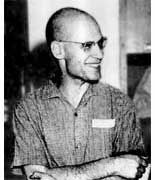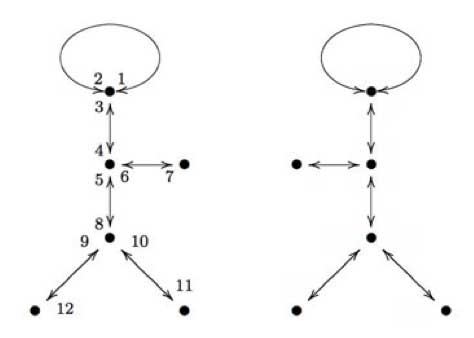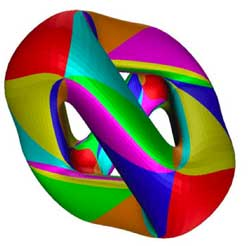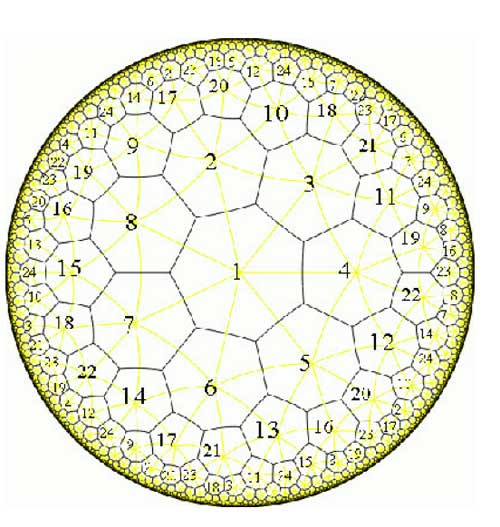# The best rejected proposal everThe Oscar in
the category The Best Rejected Research Proposal in Mathematics
(ever)
goes to … Alexander Grothendieck
for his proposal Esquisse d’un Programme, Grothendieck\’s research program from 1983, written as
part of his application for a position at the CNRS, the French
equivalent of the NSF. An English translation is
available.

Here is one of the problems discussed :
Give TWO non-trivial elements of
$Gal(\overline{\mathbb{Q}}/\mathbb{Q})$
the _absolute_
Galois group of the algebraic closure of the rational numbers
$\overline{\mathbb{Q}}$, that is the group of all
$\mathbb{Q}$-automorphisms of $\overline{\mathbb{Q}}$. One element
most of us can give (complex-conjugation) but to find any other
element turns out to be an extremely difficult task.

To get a handle on
this problem, Grothendieck introduced his _’Dessins d’enfants’_
(Children’s drawings). Recall from last session the pictures of the
left and right handed Monsieur MathieuThe left hand side drawing was associated to a map
$\mathbb{P}^1_{\mathbb{C}} \rightarrow \mathbb{P}^1_{\mathbb{C}}$ which was
defined over the field $\mathbb{Q} \sqrt{-11}$ whereas the right side
drawing was associated to the map given when one applies to all
coefficients the unique non-trivial automorphism in the Galois group
$Gal(\mathbb{Q}\sqrt{-11}/\mathbb{Q})$ (which is
complex-conjugation). Hence, the Galois group
$Gal(\mathbb{Q}\sqrt{-11}/\mathbb{Q})$ acts _faithfully_ on the
drawings associated to maps $\mathbb{P}^1_{\mathbb{Q}\sqrt{-11}} \rightarrow \mathbb{P}^1_{\mathbb{Q}\sqrt{-11}}$ which are ramified only over
the points ${ 0,1,\infty }$.

Grothendieck’s idea was to
extend this to more general maps. Assume that a projective smooth curve
(a Riemann surface) X is defined over the algebraic numbers
$\overline{\mathbb{Q}}$ and assume that there is a map $X \rightarrow \mathbb{P}^1_{\mathbb{C}}$ ramified only over the points
${ 0,1,\infty }$, then we can repeat the procedure of last time and
draw a picture on X consisting of d edges (where d is the degree
of the map, that is the number of points lying over another point of
$\mathbb{P}^1_{\mathbb{C}}$) between white resp. black points (the
points of X lying over 1 (resp. over 0)).

Call such a drawing a
‘dessin d\’enfant’ and look at the collection of ALL dessins
d’enfants associated to ALL such maps where X runs over ALL curves
defined over $\overline{\mathbb{Q}}$. On this set, there is an action
of the absolute Galois group
$Gal(\overline{\mathbb{Q}}/\mathbb{Q})$ and if this action would be
faithful, then this would give us insight into this
group. However, at that time even the existence of a map $X \rightarrow \mathbb{P}^1$ ramified in the three points ${ 0,1,\infty }$
seemed troublesome to prove, as Grothendieck recalls in his proposal

In more erudite terms, could it be true that
every projective non-singular algebraic curve defined over a number
field occurs as a possible ‚ modular curve‚ parametrising
elliptic curves equipped with a suitable rigidification? Such a
supposition seemed so crazy that I was almost embarrassed to submit
it to the competent people in the domain. Deligne when I consulted
him found it crazy indeed, but didn’t have any counterexample up
his sleeve. Less than a year later, at the International Congress in
Helsinki, the Soviet mathematician Bielyi announced exactly that result,
with a proof of disconcerting simplicity which fit into two little
pages of a letter of Deligne ‚ never, without a doubt, was such a
deep and disconcerting result proved in so few lines!

In
the form in which Bielyi states it, his result essentially says that
every algebraic curve defined over a number field can be obtained as
a covering of the projective line ramified only over the points 0,
1 and infinity. This result seems to have remained more or less
unobserved. Yet, it appears to me to have considerable importance. To
me, its essential message is that there is a profound identity
between the combinatorics of finite maps on the one hand, and the
geometry of algebraic curves defined over number fields on the
other. This deep result, together with the algebraic- geometric
interpretation of maps, opens the door onto a new, unexplored world within reach of all, who pass by without seeing it.

Belyi’s proof is indeed relatively easy
(full details can be found in the paper Dessins d’enfants on the
Riemann sphere
by Leila
Schneps). Roughly it goes as follows : as both X and the map are
defined over $\overline{\mathbb{Q}}$ the map is only ramified over
(finitely many) $\overline{\mathbb{Q}}$-points. Let S be the finite
set of all Galois-conjugates of these points and consider the polynomial

$f_0(z_0) = \prod_{s \in S} (z_0 -s) \in \mathbb{Q}[z_0]$

Now, do a
resultant trick. Consider the
polynomial $f_1(z_1) = Res_{z_0}(\frac{d f_0}{d z_0},f_0(z_0)-z_1)$ then the roots of $f_1(z_1)$ are exactly the
finite critical values of $f_0$, $f_1$ is again defined over
$\mathbb{Q}$ and has lower degree (in $z_1$) than $f_0$ in $z_1$.
Continue this trick a finite number of times untill you have constructed
a polynomial $f_n(z_n) \in \mathbb{Q}[z_n]$ of degree zero.

Composing
the original map with the maps $f_j$ in succession yields that all
ramified points of this composition are
$\mathbb{Q}$-points! Now, we only have to limit the number of
these ramified $\mathbb{Q}$-points (let us call this set T) to three.

Take any three elements of T, then there always exist integers $m,n \in \mathbb{Z}$ such that the three points go under a linear
fractional transformation (a Moebius-function associated to a matrix in
$PGL_2(\mathbb{Q})$) to ${ 0,\frac{m}{m+n},1 }$. Under the
transformation $z \rightarrow \frac{(m+n)^{m+n}}{m^m n^n}z^m(1-z)^n$ the points 0 and 1 go to 0 and
$\frac{m}{m+n}$ goes to 1 whence the ramified points of the
composition are one less in number than T. Continuing in this way we
can get the set of ramified $\mathbb{Q}$-points of a composition at
most having three elements and then a final Moebius transformation gets
them to ${ 0,1,\infty }$, done!As a tribute for this clever
argument, maps $X \rightarrow \mathbb{P}^1$ ramified only in 0,1 and
$\infty$ are now called Belyi morphisms. Here is an example of
a Belyi-morphism (and the corresponding dessin d’enfants) associated to
one of the most famous higher genus curves around : the Klein
quartic
(if you haven’t done
so yet, take your time to go through this marvelous pre-blog post by
John Baez).

One can define the Klein quartic as the plane projective
curve K with defining equation in
$\mathbb{P}^2_{\\mathbb{C}}$ given by $X^3Y+Y^3Z+Z^3X = 0$ K has
a large group of automorphism, namely the simple group of order
168 $G = PSL_2(\mathbb{F}_7) = SL_3(\mathbb{F}_2)$ It is a classical fact (see for example
the excellent paper by Noam Elkies The Klein quartic in number theory) that the quotient map $K \rightarrow K/G = \mathbb{P}^1_{\mathbb{C}}$ is ramified only in the points
0,1728 and $\infty$ and the number of points of K lying over them
are resp. 56, 84 and 24. Now, compose this map with the Moebius
transormation taking ${ 0,1728,\infty } \rightarrow { 0,1,\infty }$
then the resulting map is a Belyi-map for the Klein quartic. A
topological construction of the Klein quartic is fitting 24 heptagons
together so that three meet in each vertex, see below for the gluing
data-picture in the hyperbolic plane : the different heptagons are given
a number but they appear several times telling how they must fit
together)The resulting figure has exactly $\frac{7 \times 24}{2} = 84$ edges and the 84 points of K lying over 1 (the white points in
the dessin) correspond to the midpoints of the edges. There are exactly
$\frac{7 \times 24}{3}=56$ vertices corresponding to the 56 points
lying over 0 (the black points in the dessin). Hence, the dessin
d\’enfant associated to the Klein quartic is the figure traced out by
the edges on K. Giving each of the 168 half-edges a
different number one assigns to the white points a permutation of order
two and to the three-valent black-points a permutation of order three,
whence to the Belyi map of the Klein quartic corresponds a
168-dimensional permutation representation of $SL_2(\mathbb{Z})$,
which is not so surprising as the group of automorphisms is
$PSL_2(\mathbb{F}_7)$ and the permutation representation is just the
regular representation of this group.

Next time we will see how
one can always associate to a curve defined over
$\overline{\mathbb{Q}}$ a permutation representation (via the Belyi
map and its dessin) of one of the congruence subgroups $\Gamma(2)$ or
$\Gamma_0(2)$ or of $SL_2(\mathbb{Z})$ itself.

### Similar Posts:

This site uses Akismet to reduce spam. Learn how your comment data is processed.